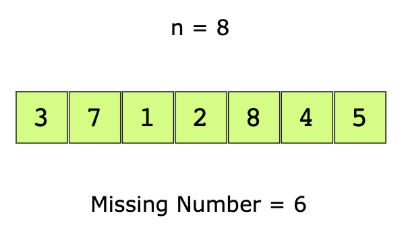# Find the Missing Number

## Problem Statement

You are given an array of positive numbers from $1$ to $n$, such that all numbers from $1$ to $n$ are present except one number, $x$. You have to find $x$. The input array is not sorted.

For example, let’s look at the below array.## Hint

• How would you calculate the sum of numbers from $1$ to $n$?

## Try it yourself

#include <iostream>#include <vector>using namespace std;int FindMissing(const vector<int>& input) {	//TODO: Write - Your - Code	return -1;}

## Solution

#include <iostream>#include <vector>#include <string>#include <numeric>using namespace std;int FindMissing(const vector<int>& input) {	// Calculate sum of all elements in the input vector	// The accumulate function can this for us	int actual_sum = accumulate(input.begin(), input.end(), 0);	int n = input.size();	// Find the sum of first n numbers using the arithmetic series sum formula,	// i.e.,​ ​(n∗(n+1))/2	int sum_of_n = (n * (n + 1)) / 2;	// The difference between sum_of_n and actual_sum, is the missing number in the	// array	return sum_of_n - actual_sum;}int main() {	vector<vector<int> > vec_list = {{0}, {3, 7, 1, 2, 0, 4, 5}, {9, 6, 4, 2, 3, 5, 7, 0, 1}};	for (int i = 0; i < vec_list.size(); i++) {		cout << i + 1 << ". Original ";		// A custom function to print the vector		PrintList(vec_list[i]);		int missing = FindMissing(vec_list[i]);		cout << "   The missing number is: " << missing << endl;		cout << "\n------------------------------------------------------------------------------------------------------\n" << endl;	}}

## Solution Explanation

### Runtime Complexity

Linear, $O(n)$.

### Memory Complexity

Constant, $O(1)$.

### Solution Breakdown

A naive solution is to simply search for every integer between 1 and n in the input array, stopping the search as soon as there is a missing number. Since the input array is not sorted, its time complexity will be $O(n^{2}$).

Can you do better than $O(n^{2}$)? Yes.

You could sort the array and then do a linear scan $O(n$) where you compare all consecutive elements to find the missing number. However, the time complexity of this algorithm is $O(n log n$) due to the time spent in sorting.

You can do better. Here is a linear, $O(n$), solution that uses the arithmetic series sum formula.

$\sum_{1}^n = \frac{n (n + 1)}{2}$

​​Here are the steps to find the missing number.

1. Find the sum of elements of all the numbers in the array. Let’s call it sum. This would require a linear scan, $O(n)$.
2. Then find the expected sum of the first $n$ numbers using the formula: $\frac{(n(n+ 1))}{2}$. Let’s call it total.
3. The difference between total and sum is the missing number in the array.

Practice problems like this and many more by checking out our Grokking the Coding Interview: Patterns for Coding Questions course!

Learn in-demand tech skills in half the time